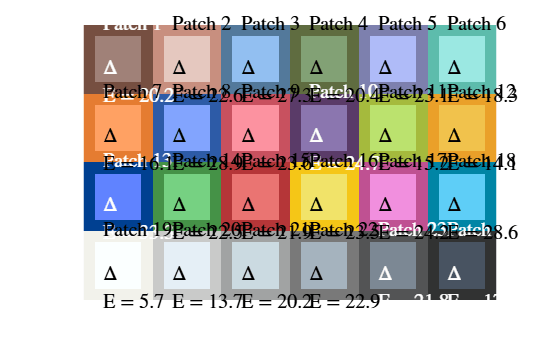# Calculate CIE94 Color Difference of Colors on Test Chart

This example shows how to calculate the color difference of measured and reference colors using the CIE94 standard.

The `measureColor` function measures colors on a test chart and calculates the color difference between measured and reference colors using the CIE76 standard. You can use the `imcolordiff` function to calculate the color difference using the CIE94 or CIEDE2000 standard.

Read an image of a ColorChecker® chart into the workspace.

`I = imread("colorCheckerTestImage.jpg");`

Create a `colorChecker` object then display the chart with ROI annotations.

```chart = colorChecker(I); displayChart(chart)```Measure the color in each color patch ROI and return the measurements in a table, `colorTable`. The color difference measurements in the `Delta_E` variable of the table follow the CIE76 standard.

`colorTable = measureColor(chart);`

On a color patch diagram, display the measured and reference colors with the corresponding CIE76 color difference superimposed on each patch.

`displayColorPatch(colorTable)`Extract the reference L*a*b* and measured RGB color values into a table.

```referenceLab = colorTable{:,["Reference_L","Reference_a","Reference_b"]}; measuredRGB = colorTable{:,["Measured_R","Measured_G","Measured_B"]};```

Convert the measured RGB colors to the L*a*b* color space, specifying a D50 white point.

`measuredLab = rgb2lab(measuredRGB,WhitePoint="d50");`

Calculate the color difference using the `imcolordiff` function, specifying that the color measurements are in the L*a*b* color space. By default, this function calculates color differences using the CIE94 standard.

`dE = imcolordiff(measuredLab,referenceLab,isInputLab=true);`

Create a new color table using the new color difference measurements.

```colorTable94 = colorTable; colorTable94{:,"Delta_E"} = dE;```

On a color patch diagram, display the measured and reference colors with the corresponding CIE94 color difference superimposed on each patch.

`displayColorPatch(colorTable94)`